# Manifold lifting: scaling MCMC to the vanishing noise regime¶

This notebook accompanies the paper Manifold lifting: scaling MCMC to the vanishing noise regime and illustrates applying the method described in the paper to a toy two-dimensional model using the Python packages Mici and SymNum.

## Set up environment¶

We first set up our environment by importing the Python objects we will need into the main namespace. We check if the notebook is running on Binder or Google Colab and set flags if so, also installing the required Python dependencies using pip if running on Colab (this is handled automatically by Binder using the requirements.txt files in the root of the repository.

In :
import os
ON_BINDER = 'BINDER_SERVICE_HOST' in os.environ

try:
import google.colab
ON_COLAB = True
except ImportError:
ON_COLAB = False

if ON_COLAB:
!pip install mici>=0.1.7 symnum>=0.1.1 arviz>=0.5

In :
import time
import inspect
from functools import partial
from itertools import product
import symnum.numpy as snp
from symnum import (
numpify, named_array, jacobian, grad,
vector_jacobian_product, matrix_hessian_product)
import numpy as np
import sympy
import matplotlib.pyplot as plt
from mpl_toolkits.mplot3d import Axes3D
import matplotlib.colors as mcolors
from ipywidgets import interact, fixed, Dropdown
from IPython.display import Javascript
import arviz
import mici
%matplotlib inline


We set some Matplotlib styling parameters to improve the appearance of plots and seed a NumPy pseudo-random number generator for reproducibility.

In :
plt.rcParams.update({
'mathtext.fontset': 'cm',
'axes.titlesize': 12,
'axes.labelsize': 12,
'xtick.labelsize': 8,
'ytick.labelsize': 8,
'legend.fontsize': 10,
'axes.linewidth': 0.5,
'lines.linewidth': 1.,
'axes.labelpad': 2.,
'figure.dpi': 100,
'figure.figsize': (6, 6),
'legend.frameon': False,
'animation.html': 'html5'
})

dens_cmap = mcolors.LinearSegmentedColormap.from_list(
'whiteblue', [np.array((1.000, 1.000, 1.000, 1.)),
np.array((0.419, 0.682, 0.840, 1.)),
np.array((0.031, 0.188, 0.420, 1.))])

seed = 20200310
rng = np.random.default_rng(seed)


At various points in this notebook we produce plots for different values of one or more parameters to illustrate how this parameter effects the plotted quantity. If this notebook is being displayed statically (e.g. directly from Github or on NBViewer) then these plots are displayed as a grid of axes. However, when the notebook is being run interactively with an active kernel (e.g. when running on Binder, Colab or locally), then we can instead use the interact function provided in ipywidgets to produce interactive plots that allow the parameters to be altered using slider widgets. To allow flexibility in covering both cases we define a helper function that creates either a static grid plot or an interactive plot. The plot type is changeable when an active kernel is available using a dropdown menu; you will also need to rerun the cell after changing the dropdown.

In :
# To always use interactive plots by default comment the first line below
# and uncomment the second
default_plot_type = 'Static' if not (ON_COLAB or ON_BINDER) else 'Interactive'
# default_plot_type = 'Interactive'

plot_type = Dropdown(value=default_plot_type, description='Plot type',
options=['Interactive', 'Static'])

def interact_or_grid(plot_function, figsize=(12, 12), subplot_kw=None, **kwargs):
display(plot_type)
grid_params = kwargs.pop('grid_params', ('y', 'σ'))
params = {k: v if plot_type.value == 'Interactive' else v
for k, v in kwargs.items()}
if plot_type.value == 'Interactive':
interact(plot_function, **params, ax=fixed(None))
else:
fig, axes = plt.subplots(
len(params[grid_params]), len(params[grid_params]),
figsize=figsize, subplot_kw=subplot_kw)
grid_param_0_vals = params.pop(grid_params)
grid_param_1_vals = params.pop(grid_params)
for (p0, p1), ax in zip(product(grid_param_0_vals, grid_param_1_vals),
axes.flat):
plot_function(
**{grid_params: p0, grid_params: p1}, **params, ax=ax)
ax.set_title(fr'${grid_params}={p0}, {grid_params}={p1}$')
fig.tight_layout()


## Problem statement¶

The problem we aim to tackle is that of inferring a latent vector $\boldsymbol{\theta} \in \Theta \subseteq \mathbb{R}^{d_{\Theta}}$ given noisy observations $\boldsymbol{y} \in \mathcal{Y} = \mathbb{R}^{d_{\mathcal{Y}}}$ generated according to a model of the form

$$\boldsymbol{y} = F(\boldsymbol{\theta}) + \sigma \boldsymbol{\eta}$$

where $F : \Theta \to \mathcal{Y}$ is the forward function, $\sigma > 0$ is the observation noise standard deviation, and $\boldsymbol{\eta} \sim \mathcal{N}(\boldsymbol{0},\mathbf{I}_{d_{\mathcal{Y}}})$ a random vector representing the additive observation noise.

We take a Bayesian approach and assume our prior beliefs about the latent vector $\boldsymbol{\theta}$ are specified by a distribution on $\Theta$ with unnormalised density $\exp(-\Phi_0(\boldsymbol{\theta}))$ with respect to the Lebesgue measure. The posterior distribution on $\Theta$ then has an (unnormalised) density $\pi^{\sigma} : \Theta \to \mathbb{R}_{\geq 0}$ defined by

$$-\log\pi^\sigma(\boldsymbol{\theta}) = \Phi_0(\boldsymbol{\theta}) + \frac{1}{2 \sigma^2} \|\boldsymbol{y} - F(\boldsymbol{\theta})\|^2.$$

Other than in special cases such as when the forward function $F$ is linear and the prior Gaussian, we cannot evaluate integrals with respect to the posterior distribution analytically and so instead need to use approximate inference methods to estimate posterior expectations. A particularly challenging regime for approximate inference methods, such as Markov chain Monte Carlo (MCMC) methods, is when the observation noise standard deviation $\sigma$ is small and each observation highly informative. As we will see this produces a posterior distribution with a challenging geometry for MCMC methods to efficiently explore.

## Toy two-dimensional model¶

As a simple example to illustrate the problem and our proposed inference approach, we use a model with $d_{\Theta} = 2$ and $d_{\mathcal{Y}} = 1$ and forward function

$$F(\boldsymbol{\theta}) = [\theta_1^2 + 3 \theta_0^2 \, (\theta_1^2 - 1)].$$

We can define this forward function in Python as follows

In :
dim_θ = 2
dim_y = 1

@numpify(dim_θ)
def forward_func(θ):
return snp.array([θ**2 + 3 * θ**2 * (θ**2 - 1)])


Here we use the numpy interface of the SymNum package to define the function, which will later allow us to use SymNum to automatically calculate derivatives. The numpify decorator generates a function which accepts and outputs NumPy arrays from the decorated function, which itself acts on symbolic array arguments. The decorator requires that we specify the dimension(s) of the array argument(s). We visualise the geometry of this forward operator by evaluating it over an equispaced grid of $\boldsymbol{\theta}$ values in $[-2, 2] \times [-2,2]$ and plotting the resulting field as a two-dimensional pseudocolour map with contours.

In :
θ_grid = np.stack(
np.meshgrid(np.linspace(-2, 2, 201), np.linspace(-2, 2, 201)))
f_grid = forward_func(θ_grid)
fig, ax = plt.subplots(figsize=(6, 5))
mesh = ax.pcolormesh(θ_grid, θ_grid, f_grid)
cset = ax.contour(θ_grid, θ_grid, f_grid, [-0.5, 0, 1, 3, 10, 20],
colors='white')
ax.clabel(cset, fmt='%.2g')
cax = fig.colorbar(mesh)
_ = cax.set_label(r'$F(\mathbf{\theta})$')
_ = ax.set(xlabel=r'$\theta_0$', ylabel=r'$\theta_1$')
fig.tight_layout()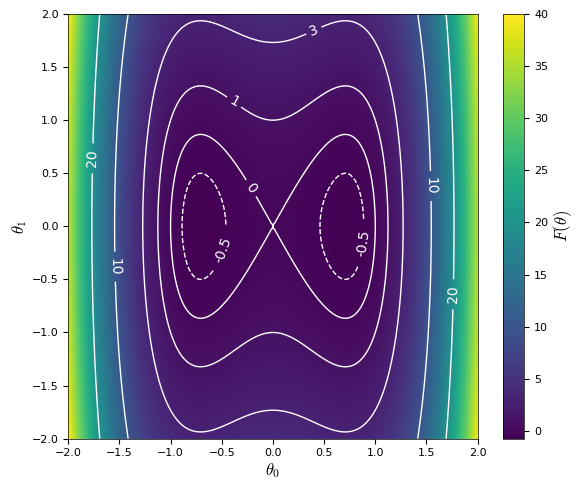We see that the level sets of $F$ form closed curves which vary in geometry for different fixed values of $F$, forming a single closed 'loop' for $F > 0$, a lemniscate for $F = 0$ and two disconnected closed loops for $F < 0$. As we will see shortly the varying geometry of the level sets of $F$ will be reflected in the geometry of the posterior distribution for different values of the observed $y$.

## Geometry of the posterior distribution¶

We assume we acquire one observation $y$ and use a standard Gaussian prior over $\boldsymbol{\theta}$, i.e. $\boldsymbol{\theta} \stackrel{\textrm{prior}}{\sim} \mathcal{N}(\boldsymbol{0}, \mathbf{I}_2)$. The goal of the inference is then to identify the set of $\boldsymbol{\theta}$ values that explain the observed $y$. Using SymNum we can define a function to evaluate the negative logarithm of the (unnormalised) posterior density, $-\log\pi^\sigma$, as follows.

In :
@numpify(dim_θ, None, None)
def neg_log_posterior_dens(θ, σ, y):
return (snp.sum(θ**2, 0) + snp.sum((y - forward_func(θ))**2, 0) / σ**2) / 2


The geometry of the resulting posterior distribution depends on the values of both $y$ and $\sigma$. Below we plot the posterior density $\pi^\sigma$ across $\boldsymbol{\theta}$ values in $[-2, 2] \times [-2, 2]$ for different $y$ and $\sigma$ values.

In :
def create_fig_if_none(ax, **fig_kw):
if ax is None:
fig, ax = plt.subplots(**fig_kw)
else:
fig = ax.figure
return fig, ax

def plot_posterior_density(σ=0.1, y=1., ax=None):
fig, ax = create_fig_if_none(ax)
ax.pcolormesh(θ_grid, θ_grid,
np.exp(-neg_log_posterior_dens(θ_grid, σ, y)),
cmap=dens_cmap)
ax.set(xlabel=r'$\theta_0$', ylabel=r'$\theta_1$')
return fig, ax

interact_or_grid(
plot_posterior_density, figsize=(12, 12),
σ=[(0.02, 1., 0.02), (0.5, 0.1, 0.02)],
y=[(-0.5, 2., 0.1), (1, 0, -0.5)])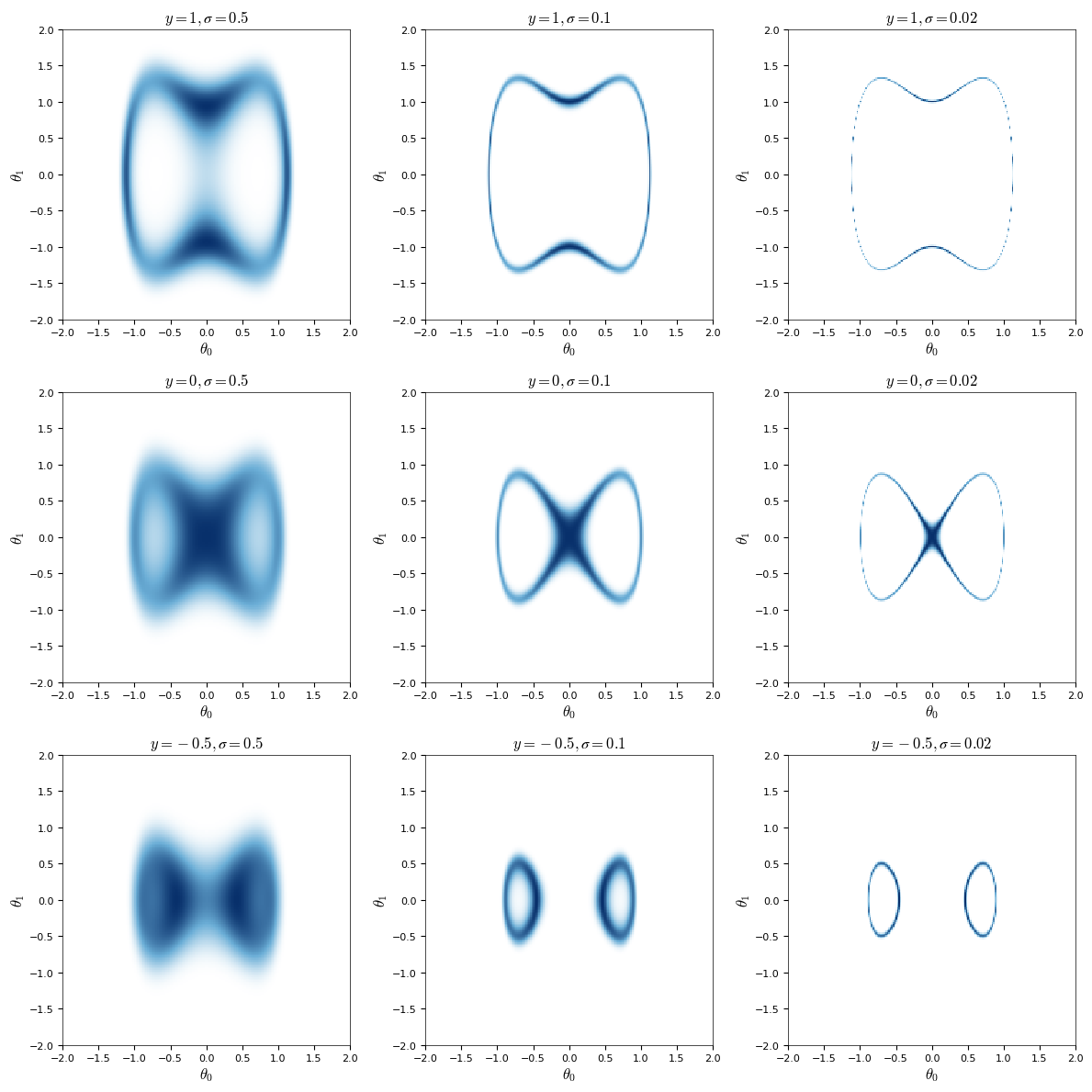We can see that for all values of $y$ as $\sigma \rightarrow 0$, the posterior distribution concentrates on a limiting manifold $\mathcal{S}= \{\boldsymbol{\theta} : F(\boldsymbol{\theta}) = y\}$, corresponding to a specific level set of $F$. For our simple test model, we can visualise $\mathcal{S}$ directly by explicitly solving $F(\boldsymbol{\theta}) = \boldsymbol{y}$ for $\theta_1$ in terms of $\theta_0$. This can be done using SymPy as in the following function (the details of how the solution is calculated are unimportant here).

In :
def split_into_integer_parts(n, m):
return [round(n / m)] * (m - 1) + [n - round(n / m) * (m - 1)]

def grid_on_interval(interval, n_points, cosine_spacing=False):
if cosine_spacing:
# Use non-linear spacing with higher density near endpoints
ts =  ((1 + np.cos(np.linspace(0, 1, n_points) * np.pi)) / 2)
else:
ts = np.linspace(0, 1, n_points)
# If open interval space over range [left + eps, right - eps]
eps = 10 * np.finfo(np.float64).eps
left = (float(interval.left) + eps if interval.left_open
else float(interval.left))
right = (float(interval.right) - eps if interval.right_open
else float(interval.right))
return left + ts * (right - left)

def solve_for_limiting_manifold(y, n_points=200, cosine_spacing=False):
assert n_points % 2 == 0, 'n_points must be even'
θ = named_array('θ', 2)
# solve F(θ) = y for θ in terms of θ
θ_1_gvn_θ_0 = sympy.solve(forward_func(θ) - y, θ)
# find interval(s) over which θ gives real θ solutions
θ_0_range = sympy.solveset(
θ_1_gvn_θ_0**2 > 0, θ, domain=sympy.Reals)
θ_0_intervals = (
θ_0_range.args if isinstance(θ_0_range, sympy.Union)
else [θ_0_range])
# create  grid of values over valid θ interval(s)
n_intervals = len(θ_0_intervals)
θ_0_grids = [
grid_on_interval(intvl, n_pt + 1, cosine_spacing)
for intvl, n_pt in zip(
θ_0_intervals,
split_into_integer_parts(n_points // 2, n_intervals))]
# generate NumPy function to calculate θ in terms of θ
solve_func = sympy.lambdify(θ, θ_1_gvn_θ_0)
manifold_points = []
for θ_0_grid in θ_0_grids:
# numerically calculate +/- θ solutions over θ grid
θ_1_grid_neg, θ_1_grid_pos = solve_func(θ_0_grid)
# stack θ and θ values in to 2D array in anticlockwise order
manifold_points.append(np.stack([
np.concatenate([θ_0_grid, θ_0_grid[-2:0:-1]]),
np.concatenate([θ_1_grid_neg, θ_1_grid_pos[-2:0:-1]])
], -1))
return manifold_points


We can verify the posterior concentrates on this limiting manifold by overlaying a plot of this manifold over the posterior density for a decreasing sequence of $\sigma$ values.

In :
def plot_limiting_manifold(y, ax=None, num_points=200):
manifold_points = solve_for_limiting_manifold(
y, num_points, cosine_spacing=True)
fig, ax = create_fig_if_none(ax)
# repeat first point to close loop by duplicating index
for component in manifold_points:
indices = np.arange(component.shape + 1) % component.shape
line, = ax.plot(component[indices, 0],
component[indices, 1], '-', color='C1', lw=1.)
return fig, ax, line

def plot_posterior_density_and_limiting_manifold(σ=0.1, y=1, ax=None):
fig, ax = plot_posterior_density(σ, y, ax)
plot_limiting_manifold(y=y, ax=ax)
return fig, ax

interact_or_grid(
plot_posterior_density_and_limiting_manifold, figsize=(12, 12),
σ=[(0.02, 1.0, 0.02), (0.5, 0.1, 0.02)],
y=[(-0.5, 2., 0.1), (1, 0, -0.5)])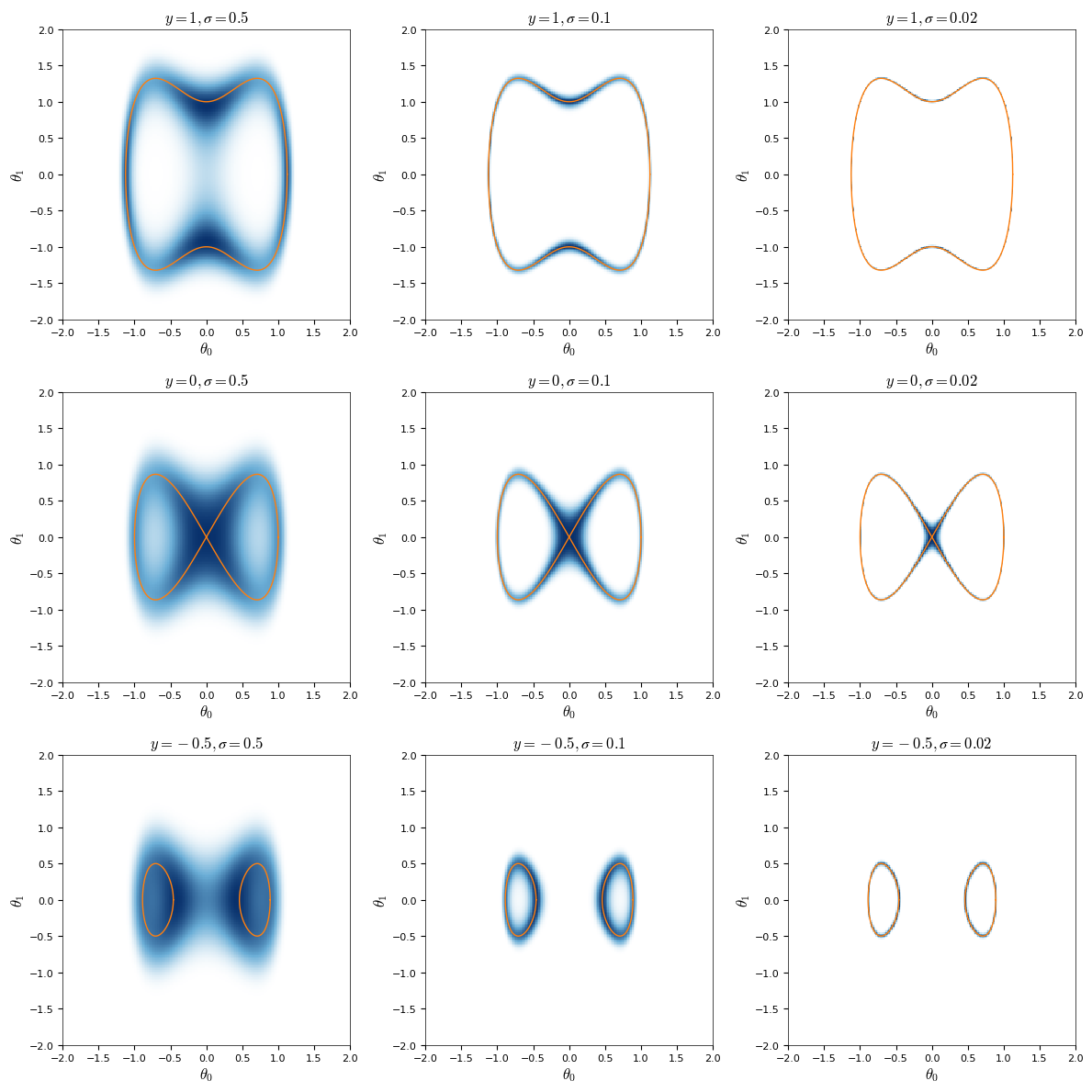The concentration of the posterior on to this lower dimensional manifold $\mathcal{S}$ makes the posterior distribution for small $\sigma$ a challenging regime for MCMC methods to operate in, with proposed moves with a non-negligible component in directions normal to the manifold having very low probability of acceptance if they start at a point in a high density region.

## Posterior density gradient field¶

MCMC methods often exploit derivative information to improve sampling efficiency. The simplest approach is to directly use the gradient of the log target density to guide proposed moves in the latent space $\Theta$ as in the Metropolis adjusted Langevin algorithm (MALA) and Hamiltonian Monte Carlo (HMC). We can construct a function to evaluate the gradient of the (negative) log posterior density using the grad operator from SymNum as follows.

In :
grad_neg_log_posterior_dens = grad(neg_log_posterior_dens)


Using this function we can now visualise the gradient vector field across the latent space $\Theta$. Below we use the Matplotlib quiver plot function to overlay the (log posterior density) gradient field over the posterior density for different $\sigma$ values. As the magnitude of the gradients vary significantly we normalise the (non-zero) gradient vectors to unit length to make the pattern of the local directions of the gradients clearer.

In :
def plot_posterior_density_and_grad_field(σ=0.1, y=1, ax=None):
fig, ax = plot_posterior_density_and_limiting_manifold(σ, y, ax)
θ_grid_grad = θ_grid[:, ::10, ::10]
grad_field = -grad_neg_log_posterior_dens(θ_grid_grad, σ, y)
grad_field_norm = np.sum(grad_field**2, 0)**0.5
grad_field[:, grad_field_norm >= 1e-8] /= grad_field_norm[grad_field_norm >= 1e-8]
ax.quiver(*θ_grid_grad, *(grad_field), angles='xy')
return fig, ax

interact_or_grid(
plot_posterior_density_and_grad_field, figsize=(12, 4),
σ=[(0.02, 1., 0.02), (0.5, 0.1, 0.02)], y=[(-0.5, 2., 0.1), (1,)])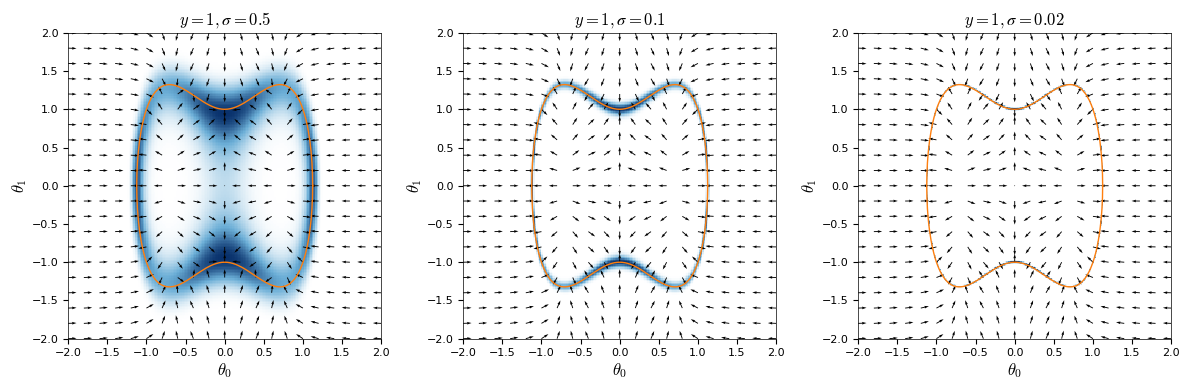We can see that for points far from the bulk of the posterior mass a fictitious particle moving under the action of a force defined by the negative log density gradient field will be pushed towards the regions of high posterior density. In regions of high posterior density however, the gradient field is close to normal to the limiting manifold. For HMC proposals which are based on discretising these gradient-based dynamics with a numerical integrator, a simulated particle trajectory starting near to the high density region will be pushed in a direction normal to (and towards) the manifold by the strong gradients in that direction. The momentum of the particle will mean that it will overshoot across limiting manifold before being pushed back in the opposite direction, leading to a characteristic high frequency oscillation back and forth across the limiting manifold. If the integrator time step used to discretise the dynamics is too large the integration of this high frequency oscillation will become unstable and diverge, with the final proposed point ending up very far from the high density region and so with a vanishingly small acceptance probability. As the 'width' of the high density region around the limiting manifold and magnitude of the log density gradients scale with $\sigma$ the integrator step size needs to be made smaller as $\sigma \to 0$ to avoid this issue.

## Hamiltonian Monte Carlo using Mici¶

To make the problems in choosing an appropriate step size for HMC as $\sigma \to 0$ more concrete, we now try applying HMC to our toy problem using Mici. Mici defines a high-level interface for construction Hamiltonian dynamics based MCMC methods, including both the standard HMC method on a Euclidean space which we will use here, and more advanced variants which involve simulating Hamiltonian dynamics on a manifold as we will see in the subsequent sections.

Mici uses a modular design to allow a wide range of MCMC methods to be constructed by using different combinations of the objects involved in sampling. The mici.systems module defines various Hamiltonian system classes which are used to represent the Hamiltonian system associated with the target distribution to be sampled, and which encapsulate the various functions associated with the system. Initially we will be using the EuclideanMetricSystem class which corresponds to a Hamiltonian system where the latent space is equipped with a metric with a fixed positive definite matrix representation (often termed the mass matrix). By default the class uses an identity matrix for the metric representation, corresponding to the standard Euclidean metric, and we will use this default in the experiments here.

If we denote the auxiliary momentum variable as $\boldsymbol{p} \in \mathbb{R}^{d_\Theta}$ and $U(\boldsymbol{\theta}) = -\log\pi^\sigma(\boldsymbol{\theta})$ then the Hamiltonian for the system is

$$H(\boldsymbol{\theta},\boldsymbol{p}) = U(\boldsymbol{\theta})+ \frac{1}{2}\boldsymbol{p}^\mathsf{T}\boldsymbol{p},$$

and the corresponding Hamiltonian dynamics are described by the ordinary differential equation (ODE) system

$$\dot{\boldsymbol{\theta}} = \boldsymbol{p}, \quad \dot{\boldsymbol{p}} = -\nabla U(\boldsymbol{\theta}).$$

Eliminating $\boldsymbol{p}$ by taking the time-derivative of the first equation we have that $\ddot{\boldsymbol{\theta}} = -\nabla U(\boldsymbol{\theta})$, which by comparing with Newton's second law we can see the simulated dynamics correspond to those of a particle acting under a force field defined by the negative gradient of a potential energy function $U$.

The two key arguments to the EuclideanMetricSystem class initialiser are neg_log_dens and grad_neg_log_dens, corresponding respectively to a function evaluating the negative log density of the target distribution $U$ and a function evaluating the gradient of this negative log density $\nabla U$. Both functions should take a single array argument, corresponding to the position component of the chain state (for now this is directly equivalent to the original latent vector $\boldsymbol{\theta}$). The neg_log_dens function should return a single scalar value. The grad_neg_log_dens function should either return a single one-dimensional array corresponding to the evaluated gradient or a 2-tuple with the first element being the gradient array and the second element a scalar corresponding to the value of neg_log_dens function at the passed position value. This option is provided as typically when using reverse-mode algorithmic differentiation the value of a function is evaluated in the forward pass through the computational graph before evaluating the gradient in a backwards pass, and by returning both the gradient and value and caching the value, Mici can avoid subsequent separate evaluation of the neg_log_dens function.

The SymNum grad operator provides an option to also return the value of the function by passing the return_aux=True keyword argument.

In :
grad_and_val_neg_log_posterior_dens = grad(
neg_log_posterior_dens, return_aux=True)


We now construct a EuclideanMetricSystem instance using the neg_log_posterior_dens and grad_and_val_neg_log_posterior_dens functions. To meet the requirement of the neg_log_dens and grad_neg_log_dens function arguments accepting only a single array argument, we use the functools.partial function to produce a partial function with the arguments σ and y and fixed.

In :
σ = 0.1
y = 1

system = mici.systems.EuclideanMetricSystem(
neg_log_dens=partial(neg_log_posterior_dens, σ=σ, y=y),
grad_neg_log_dens=partial(grad_and_val_neg_log_posterior_dens, σ=σ, y=y))


As mentioned previously system objects in Mici encapsulate various model specific functions such as the Hamiltonian and its derivatives. Below we print all the 'public' attributes (i.e. those not prefixed with an underscore) of the system object we just created and the first line of their docstrings.

In :
def print_attributes(obj):
for attr in dir(obj):
if attr != '_':
print(f'{attr}: {inspect.getdoc(getattr(obj, attr)).splitlines()}')

print_attributes(system)

dh1_dpos: Derivative of h1 Hamiltonian component with respect to position.
dh2_dmom: Derivative of h2 Hamiltonian component with respect to momentum.
dh2_flow_dmom: Derivatives of h2_flow flow map with respect to input momentum.
dh_dmom: Derivative of Hamiltonian with respect to momentum.
dh_dpos: Derivative of Hamiltonian with respect to position.
grad_neg_log_dens: Derivative of negative log density with respect to position.
h: Hamiltonian function for system.
h1: Hamiltonian component depending only on position.
h1_flow: Apply exact flow map corresponding to h1 Hamiltonian component.
h2: Hamiltonian component depending on momentum and optionally position.
h2_flow: Apply exact flow map corresponding to h2 Hamiltonian component.
metric: Matrix representing identity operator on a vector space.
neg_log_dens: Negative logarithm of unnormalized density of target distribution.
sample_momentum: Sample a momentum from its conditional distribution given a position.


Most of the system methods listed above take a mici.states.ChainState instance as their first (or only) argument. A ChainState object in Mici encapsulates the current state of a Hamiltonian system which is minimally specified by a position (pos, a 1D array), momentum (mom, a 1D array) and integration 'direction' (dir, either +1 or -1) corresponding to whether the Hamiltonian dynamics are currently being simulated forward (dir=1) or backwards (dir=-1) in time. In addition to these variables, a ChainState instance may also contain cached values of quantities deterministically derived from these variables by a Hamiltonian system object - for example the current Hamiltonian value or its derivatives. By caching these values Mici ensures potentially expensive to evaluate functions are only evaluate once for each value of the state variables they depend on.

In the cell below we construct a ChainState instance with the position component (here corresponding to $\boldsymbol{\theta}$) sampled from the standard normal prior on $\Theta$. Rather than set the momentum in the chain state initialiser we use the system.sample_momentum method to independently sample a momentum from its conditional distribution given the position; although in this case as the momentum is independent of the position and has a standard normal distribution we could easily sample the momentum directly, for some of the systems we will encounter subsequently the momentum distribution will be position-dependent and so this approach is more general.

In :
state = mici.states.ChainState(
pos=rng.standard_normal(dim_θ), mom=None, dir=1)
state.mom = system.sample_momentum(state, rng)


In addition to the system and state objects, another key class of Mici objects are the integrator classes in the mici.integrators module. For the simple EuclideanMetricSystem we are using here, the LeapfrogIntegrator is an appropriate choice of integrator, for this system corresponding to the standard Störmer-Verlet or leapfrog integrator for separable Hamiltonian systems. The LeapfrogIntegrator initialiser takes as arguments a Hamiltonian system instance and a floating point step_size parameter corresponding to the time-step used in the numerical discretisation of the Hamiltonian dynamics.

In :
integrator = mici.integrators.LeapfrogIntegrator(system, step_size=0.05)


The key method of integrator objects is the integrator.step method which takes as argument a ChainState instance and returns a new ChainState instance corresponding to the system state simulated one time-step forward (if state.dir = 1) or backward (if state.dir = -1) in time under the Hamiltonian dynamics associated with the system. Below we iteratively apply integrator.step twice before reversing the integration direction and integrating a further two steps. The state returns to the exactly the same position and momentum values due to the time-reversibility of the leapfrog integrator.

In :
n_step = 2
state_init = state.copy()
display(state)
for s in range(n_step):
state = integrator.step(state)
display(state)
state.dir *= -1
display(state)
for s in range(n_step):
state = integrator.step(state)
display(state)
state.dir *= -1
assert (np.allclose(state.pos, state_init.pos) and
np.allclose(state.mom, state_init.mom))

ChainState(
pos=[-0.329414   -1.46108452],
mom=[-0.98537164  0.25382918],
dir=1)
ChainState(
pos=[-0.54164252 -1.13807594],
mom=[-3.13412876  4.62955666],
dir=1)
ChainState(
pos=[-0.64282688 -0.99812886],
mom=[-0.78410153 -0.82501368],
dir=1)
ChainState(
pos=[-0.64282688 -0.99812886],
mom=[-0.78410153 -0.82501368],
dir=-1)
ChainState(
pos=[-0.54164252 -1.13807594],
mom=[-3.13412876  4.62955666],
dir=-1)
ChainState(
pos=[-0.329414   -1.46108452],
mom=[-0.98537164  0.25382918],
dir=-1)

The final key group of Mici objects are the sampler classes in the mici.samplers module. A sampler object uses a Hamiltonian system and associated integrator to simulate Markov chains which leave the target distribution represented by the system invariant. The simplest sampler is the StaticMetropolisHMC class which corresponds to the Hybrid Monte Carlo algorithm proposed by Duane, Kennedy, Pendleton and Roweth (1987): each new state is proposed by integrating the dynamic forward a fixed number of steps and accepting or rejecting the move from the initial to final state on the trajectory in a Metropolis accept step. These 'integration' chain transitions in which the position and momentum components are jointly updated are alternated with transitions in which only the momentum is updated, with the default being to independently resample the momentum from its conditional distribution given the current position.

In addition to the StaticMetropolisHMC class, Mici also provides other sampler classes such as the RandomMetropolisHMC class which instead integrates the state a randomly sampled rather than fixed number of steps in each integration transition, and the DynamicMultinomialHMC class which uses a heuristic 'no-U-turn' criterion to dynamically determine how many integration steps to take (and uses a multinomial scheme to sample the new state from the whole recursively generated trajectory). As an example we construct a StaticMetropolicHMC sampler by passing the previously created system, integrator and rng (random number generator) objects as arguments to the class initialiser and setting the fixed number of integration steps to n_step=20.

In :
sampler = mici.samplers.StaticMetropolisHMC(system, integrator, rng, n_step=20)


The two key methods of a sampler object are sample_chain and sample_chains, with the former sampling a single Markov chain from an initial state and the latter multiple Markov chains from a list of initial states. The sample_chain method returns a tuple of three objects:

• final_state: State of chain after final iteration. May be used to resume sampling a chain by passing as the initial state to a new sample_chain call.
• traces: Dictionary of chain trace arrays. Values in dictionary are arrays of variables outputted by trace functions in trace_funcs argument to sample_chain with leading dimension of array corresponding to the sampling (chain iteration) index. By default trace_funcs consists of a single function returning the position component of the state and so traces contains a single entry with key pos.
• chain_stats: Dictionary of chain statistics. Values in dictionary are arrays of chain statistic values with the leading dimension of each array corresponding to the sampling (chain iteration) index. The key for each value is a string description of the corresponding statistic - for example the per-iteration acceptance probability statistics are stored under the key accept_prob.

The sample_chains method also returns a tuple of three objects but with each object now contain lists of per-chain states / arrays (see docstring of sample_chains for more details).

As well as an init_state argument specifying the initial chain state (either as a ChainState instance or a 1D NumPy array specifying only the position component with the momentum component then sampled independently using system.sample_momentum) the other required argument to the sample_chain method is n_sample, the number of chain samples to generate. Below we call sample_chain to sample a chain of 2000 iterations starting from the chain state state constructed previously. During sampling by default Mici displays a progress bar showing how many chain iterations have been completed so far, the expected time to completion and running averages of chain statistics such as the acceptance probability of Metropolis transitions (the statistics to be monitored can be altered by setting the monitor_stats argument to sample_chains, see docstring for details).

In :
final_state, trace, stats = sampler.sample_chain(n_iter=2000, init_state=state)

2000/2000 [00:01<00:00, 1194.38it/s, accept_stat=0.662]
/tmp/symnum_grad_neg_log_posterior_dens_module_gt_zqfd5.py:8: RuntimeWarning: overflow encountered in double_scalars
/tmp/symnum_grad_neg_log_posterior_dens_module_gt_zqfd5.py:9: RuntimeWarning: overflow encountered in double_scalars
/home/matt/miniconda3/envs/man-lift/lib/python3.7/site-packages/mici/systems.py:111: RuntimeWarning: invalid value encountered in subtract
state.mom -= dt * self.dh1_dpos(state)
/tmp/symnum_grad_neg_log_posterior_dens_module_gt_zqfd5.py:2: RuntimeWarning: overflow encountered in double_scalars
/tmp/symnum_grad_neg_log_posterior_dens_module_gt_zqfd5.py:5: RuntimeWarning: overflow encountered in double_scalars
/tmp/symnum_grad_neg_log_posterior_dens_module_gt_zqfd5.py:4: RuntimeWarning: overflow encountered in double_scalars
/home/matt/miniconda3/envs/man-lift/lib/python3.7/site-packages/mici/systems.py:255: RuntimeWarning: overflow encountered in matmul
return 0.5 * state.mom @ self.dh2_dmom(state)


We see that a series of numerical warnings are produced - specifically due to numerical overflow and invalid (NaN) values being encountered in some operations. These warnings are useful inasmuch that they indicate there is some numerical issue being encountered while sampling - specifically as we will see shortly that the numerical integration of the Hamiltonian dynamics is unstable at the step size being used - however for long chains the flood of warning messages can be annoying so we instruct NumPy to ignore these warnings subsequently.

In :
_ = np.seterr(invalid='ignore', over='ignore')


If we plot the trace of the position component of the chain state over the corresponding target posterior density we see further evidence that the HMC sampler is not performing well here as the samples are concentrated in only one portion of the loop-like region of high posterior probability, with in particular the chain seeming to have been unable to enter the two narrower 'legs' of the loop.

In :
fig, ax = plot_posterior_density(σ=σ, y=y)
ax.plot(*trace['pos'].T, '.', ms=2, color='C1')

Out:
[<matplotlib.lines.Line2D at 0x7f6cacd89490>]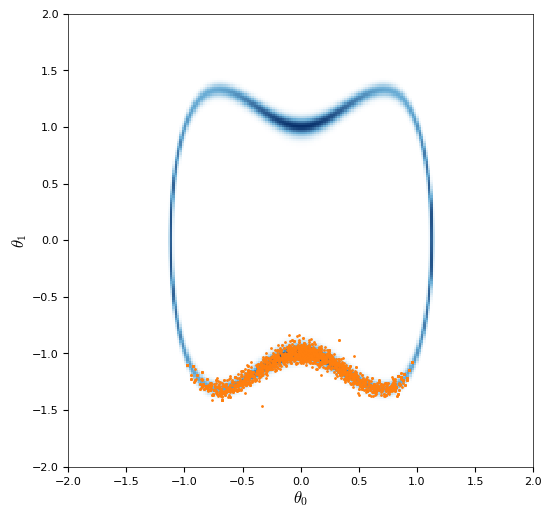While the sampler classes are the intended interface for generating chains in Mici, for the purpose of understanding the issues being encountered in running HMC in our illustrative example, we will directly use an integrator object to simulate and plot the Hamiltonian trajectories being used to propose new states in the HMC chain. The function simulate_proposal in the cell below implements a basic Metropolis-adjusted HMC algorithm with a fixed number of integration steps n_step per proposal (i.e. equivalent to the transitions implemented by the StaticMetropolisHMC sampler class). Rather than return just the next chain state (either the final state in the simulated trajectory if the move is accepted or the previous state if rejected) the simulate_proposal function here returns the full simulated trajectory and a boolean flag indicating whether the proposed move was accepted, so as to allow the proposal to be visualised. As well as rejecting due to the Metropolis accept step, in the implementation below rejections may also be triggered if the integrator diverges. This is indicated by the change in Hamiltonian over the trajectory (which should be zero under the exact dynamics) exceeding a threshold, in which case the trajectory is terminated early. In the manifold MCMC methods discussed later rejections may also be triggered by divergence of the numerical solvers involved in computing the individual (implicit) integrator steps, with this resulting in the same behaviour of terminating the trajectory early and rejecting the proposal.

In :
def simulate_proposal(state, system, integrator, rng, n_step=10):
state.mom = system.sample_momentum(state, rng)
θ_traj = np.full((n_step + 1, dim_θ), np.nan)
θ_traj = state.pos[:dim_θ]
h_init = system.h(state)
try:
for s in range(n_step):
state = integrator.step(state)
if abs(system.h(state) - h_init) > 1000:
raise mici.errors.HamiltonianDivergenceError
θ_traj[s + 1] = state.pos[:dim_θ]
accepted = rng.uniform() < np.exp(h_init - system.h(state))
except mici.errors.Error as e:
accepted = False
return θ_traj, accepted


We also define a helper function to plot a proposed trajectory, with the initial state shown by a larger marker and the trajectory coloured green if accepted and red if not.

In :
def plot_trajectory(ax, θ_traj, accepted):
ax.plot(θ_traj[:, 0], θ_traj[:, 1], '-o', ms=2, lw=1,
color=('limegreen' if accepted else 'red'))
ax.plot(θ_traj[0, 0], θ_traj[0, 1], 'o',
color=('limegreen' if accepted else 'red'))


Using the two helper functions we just defined, we now plot simulated HMC proposals for various values of the noise standard deviation $\sigma$ and integrator step size $\epsilon$ on our running toy example. The proposals are initialised at series of points on the limiting manifold $\mathcal{S}$ and so are reflective of proposals for chains which have already converged to the high probability region of the target posterior distribution.

In :
def plot_hmc_proposals(σ=0.1, ϵ=0.1, y=1, n_step=20, n_particle=4, ax=None):
system = mici.systems.EuclideanMetricSystem(
neg_log_dens=partial(neg_log_posterior_dens, σ=σ, y=y),
grad_neg_log_dens=partial(grad_neg_log_posterior_dens, σ=σ, y=y))
integrator = mici.integrators.LeapfrogIntegrator(system, step_size=ϵ)
states = [mici.states.ChainState(pos=θ, mom=None, dir=1)
for θ in np.concatenate(solve_for_limiting_manifold(y, n_particle))]
fig, ax = plot_posterior_density(σ, y, ax)
rng = np.random.default_rng(seed)
for state in states:
plot_trajectory(ax, *simulate_proposal(
state, system, integrator, rng, n_step))

interact_or_grid(
plot_hmc_proposals,
σ=[(0.02, 1., 0.02), (0.5, 0.1, 0.02)],
ϵ=[(0.02, 1., 0.02), (0.5, 0.1, 0.02)],
y=[(-0.5, 2.), y],
n_step=[(10, 100, 10), 10],
n_particle=[(4, 10, 2), 4],
grid_params=('ε', 'σ')
)